Next: Maxwell-Boltzmann Statistics Up: Quantum Statistics Previous: Photon Statistics

# Bose-Einstein Statistics

Let us now consider Bose-Einstein statistics. The particles in the system are assumed to be massive, so the total number of particles,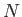, is a fixed number.

Consider the expression (8.21). For the case of massive bosons, the numbers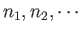assume all values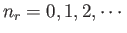for each, subject to the constraint that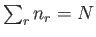. Performing explicitly the sum over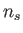, this expression reduces to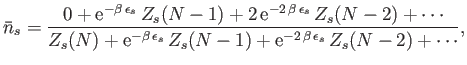(8.41)

where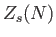is the partition function forparticles distributed over all quantum states, excluding state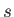, according to Bose-Einstein statistics [cf., Equation (8.23)]. Using Equation (8.28), and the approximation (8.29), the previous equation reduces to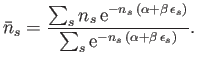(8.42)

Note that this expression is identical to (8.35), except that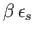is replaced by. Hence, an analogous calculation to that outlined in the previous section yields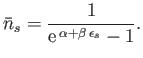(8.43)

This is called the Bose-Einstein distribution. Note that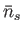can become very large in this distribution. The parameter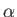is again determined by the constraint on the total number of particles: that is,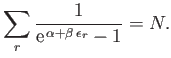(8.44)

Equations (8.20) and (8.30) can be integrated to give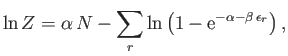(8.45)

where use has been made of Equation (8.43).

Note that photon statistics correspond to the special case of Bose-Einstein statistics in which the parametertakes the value zero, and the constraint (8.44) does not apply.Next: Maxwell-Boltzmann Statistics Up: Quantum Statistics Previous: Photon Statistics
Richard Fitzpatrick 2016-01-25